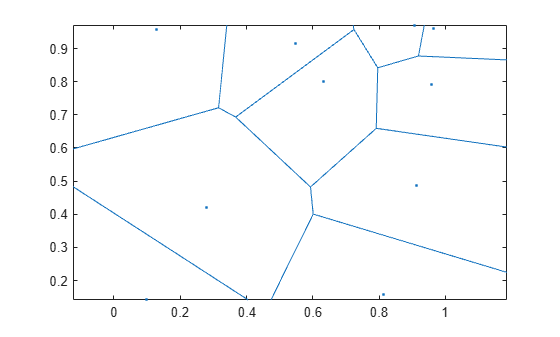# voronoi

Voronoi diagram

## Syntax

``voronoi(x,y)``
``voronoi(x,y,T)``
``voronoi(TO)``
``[vx,vy] = voronoi(___)``
``h = voronoi(___)``

## Description

example

````voronoi(x,y)` plots the bounded cells of the Voronoi diagram for the 2-D points in vectors `x` and `y`.```
````voronoi(x,y,T)` uses the Delaunay triangulation `T` to plot the Voronoi diagram.```
````voronoi(TO)` uses the `delaunayTriangulation` object `TO` to plot the Voronoi diagram.```
````[vx,vy] = voronoi(___)` returns the 2-D vertices of the Voronoi edges.```
````h = voronoi(___)` returns a graphics array of two line object handles representing the points and edges of the diagram.```

## Examples

collapse all

Create two vectors containing the coordinates of 10 2-D points, and plot the Voronoi diagram.

```rng default; x = rand([1 10]); y = rand([1 10]); voronoi(x,y) axis equal```## Input Arguments

collapse all

x-coordinates, specified as a column vector.

y-coordinates, specified as a column vector.

Delaunay triangulation, specified as a 3-column matrix. Each row of `T` contains the row indices of the input points that define a triangle in the triangulation.

Constrained Delaunay triangulation object, specified as a `delaunayTriangulation` object.

## Output Arguments

collapse all

x-coordinates of Voronoi edges, returned as a column vector.

y-coordinates of Voronoi edges, returned as a column vector.

Graphics array, returned as an array of two line object handles representing the points and edges of the diagram.

collapse all

### Voronoi Diagram

Given a point in a set of coplanar points, you can draw a boundary around it that includes all points closer to it than to any other point in the set. This boundary defines a single Voronoi polygon. The collection of all Voronoi polygons for every point in the set is called a Voronoi diagram.

## Version History

Introduced before R2006a